Courses

# Special Theory Of Realativity MCQ Level – 1

## 10 Questions MCQ Test Topic wise Tests for IIT JAM Physics | Special Theory Of Realativity MCQ Level – 1

Description
This mock test of Special Theory Of Realativity MCQ Level – 1 for Physics helps you for every Physics entrance exam. This contains 10 Multiple Choice Questions for Physics Special Theory Of Realativity MCQ Level – 1 (mcq) to study with solutions a complete question bank. The solved questions answers in this Special Theory Of Realativity MCQ Level – 1 quiz give you a good mix of easy questions and tough questions. Physics students definitely take this Special Theory Of Realativity MCQ Level – 1 exercise for a better result in the exam. You can find other Special Theory Of Realativity MCQ Level – 1 extra questions, long questions & short questions for Physics on EduRev as well by searching above.
QUESTION: 1

### A positive kaon (k+) has a rest mass of 494 MeV/c2 whereas a proton has a rest mass of 938 MeV/c2. If a koan has a total energy that is equal to the proton rest energy, speed of the kaon is most nearly :

Solution: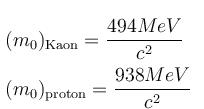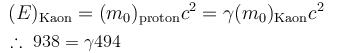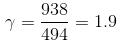On solving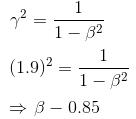The correct answer is: 0.85 c

QUESTION: 2

### A car of rest length 5 meters passes through a garage rest length 4 meters. Due to the relativistic Lorentz contraction, the car is only 3 meters long in the garage’s rest frame. There are doors on both ends of the garage, which open automatically when the front of the car reaches them and close automatically when the rear passes them. The opening or closing of each door requires a negligible amount of time. The velocity of the car in the garage’s rest frame is

Solution:

Length contraction: L0
with γ = 1/(1 − v2/c2)½

Given for the car, L0 = 5 m and = 3 m

γ = L0/L = 5/3 = 1/(1 − v2/c2)½
γ² = 25/9 = 1/(1 − v2/c2)
1 − v²/c² = 9/25
v²/c² = 1 − 9/25 = 16/25
4/c = 0.8c

QUESTION: 3

### The mean life of a meson is 2 x 10-8 sec. Calculate the mean life of a meson moving with a velocity 0.8c.

Solution:

If t0 be the mean life of meson in its own frame of reference and t  that measured by an observer in stationary frame, then according to the time dilation effect.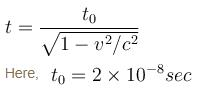v = 0.8c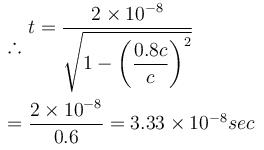The correct answer is: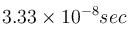QUESTION: 4

Two observers O and O' observe 2 events, A and B. The observers have a constant relative speed of 0.8c. In the units such that the speed of light is 1, observer O obtained the following coordinates.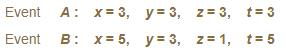What is the length of the space time interval between these 2 events, as measured by O' ?

Solution: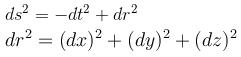= 4 + 0 + 4 = 8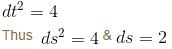QUESTION: 5

The ultraviolet Lyman α line of hydrogen with wavelength 121.5 nm   is emitted by an astronomical object. An observer on earth measures the wavelength of the light received from the object to be 607.5 nm. The observer can conclude that the object is moving with a radial velocity of :

Solution:

Since the light received has a higher wavelength than the incident wavelength, one concludes that this is a red shift, that the object is moving away from the Earth.

where λ0 is the wavelength of the source.
The doppler effect equation gives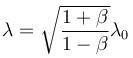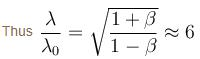Solving this one gets β = 35/37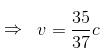v = 2.8 × 108 m/s
away from the Earth.

The correct answer is: 2.8 × 108 m/s away from Earth

QUESTION: 6

A particle leaving a cyclotron has a total relativistic energy of 10 GeV and a relativistic momentum of 8 GeV. What is the rest mass of this particle?

Solution:

Total energy = 10 GeV
p = 8 GeV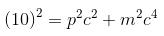In units in which c = 1 option (d)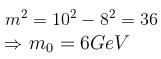The correct answer is: 6 GeV/c2

QUESTION: 7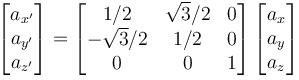The matrix shown above transforms the components of a vector in one coordinate frame S  to the components of the same vector in a second coordinate frame S'. This matrix represents a rotation of the reference frame S  by :

Solution:

The form of the matrix show that the rotation is about the z-axis

since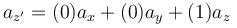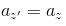since the z component does not change
∴  rotation about the z axis

Now, the standard from of the rotation matrix about z axis is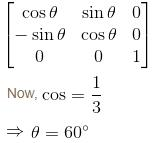QUESTION: 8

A car of rest length 5m  passes through a garage of rest length 4m. Due to the relativistic lorentz contraction, the car is only 3 long in length in the garage’s rest frame. There are doors on both ends of the garage, which open automatically when the front of the car reaches them and close automatically when the rear passes them. The opening or closing of the doors requires a negligible amount of time.

Which of the following is the best response to the question : “was the car ever inside the closed garage”

Solution:

Explanation : The order of the door opening depends on which reference frame one is in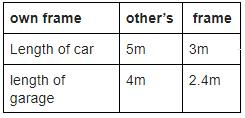So, the car fits in the garage’s frame (the car is 3m  and the garage is 4m). But in its own frame, the car is much longer to fit in the garage (car is 5m  and the garage is only 2.4m)

Both the frames give different answers.

There is no unique answer, as the order of door openings and closings depends on the reference frame.

QUESTION: 9

What is the length of a metre stick moving parallel to its length when its mass is 3/2 times of its rest mass.

Solution:

The mass of the rod is 3/2 times its rest mass, that is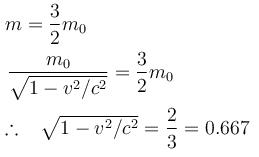The length of meter stick moving parallel to its length, according to length contraction formula.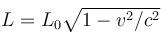L0 = 1m
L = 1 × 0.667 = 0.67m
The correct answer is: 0.67 m

QUESTION: 10

A car of rest length 5m  passes through a garage of rest length 4m. Due to the relativistic lorentz contraction, the car is only 3m  long in length in the garage’s rest frame. There are doors on both ends of the garage, which open automatically when the front of the car reaches them and close automatically when the rear passes them. The opening or closing of the doors requires a negligible amount of time.

The velocity of the car in the garage’s rest frame is

Solution:

Things moving relative to a particular frame, appear shorter in that frame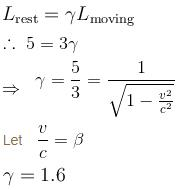⇒  β is slightly more than  0.75 c
The correct answer is: 0.8 c